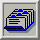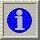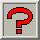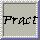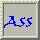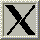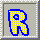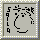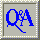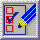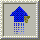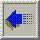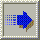##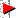3.17. Comparison of Various Algorithms

From Glassner (1990)

Here is a comparison of the computational complexity of some of the algorithms we have looked at:

 ``` Memory Search for Representatives Mapping Picture Quality Uniform Quant. 0 O(K) O(N) bad Popularity O(D) O(N + D.log(K)) >O(N) depends algorithm on data Median Cut O(D) O(N + D.log(K)) O(N.log(K)) good Octree Quant. O(K) O(N) O(N) good ```
where
N is the number of pixels of the original image. If the image is run-length encoded, N can also be the number of runs of the image.
K is the number of representatives, that is, the size of the colour look-up table.
D is the number of different colours in the original image.